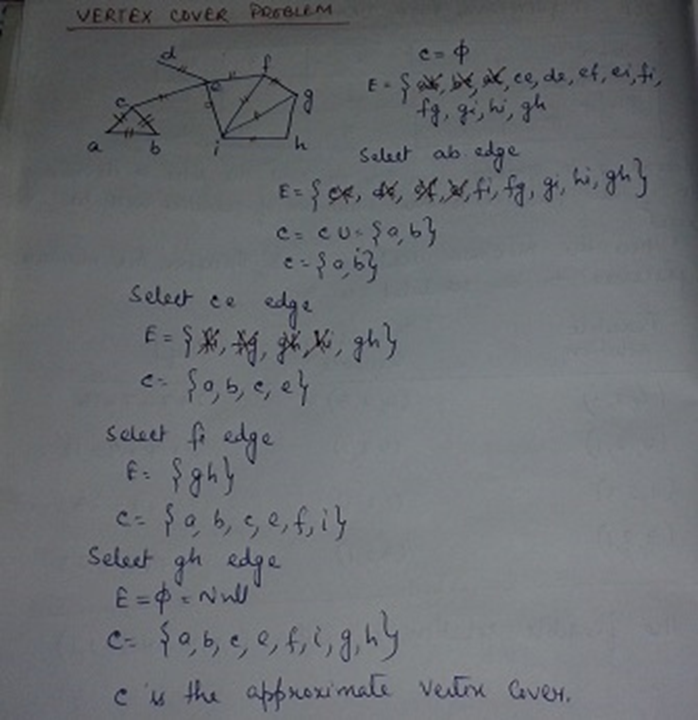Question:
Published on: 10 August, 2022

Write short notes on

• Vertex cover problem
• Strassen’s matrix multiplication
• Approximation algorithm and its uses

Vertex cover problem:

A vertex cover of an undirected graph is a subset of its vertices such that for every edge (u, v) of the graph, either ‘u’ or ‘v’ is in vertex cover.   Although the name is Vertex Cover, the set covers all edges of the given graph.  strong>Given an undirected graph, the vertex cover problem is to find minimum size vertex cover. It is a known   NP Complete problem, i.e., there is no polynomial time solution for this unless P = NP. There are approximate polynomial time algorithms to solve the problem though. Following is a simple approximate algorithm.

Approximation algorithm for vertex cover

{

C=null

While E!=null

{

Pick any edge (uv)€E

C=CU{u,v}

Delete all edges incident to either u and v

}

Return C as approximate vertex cover.

}Strassen’s matrix multiplication:

It is the method of square matrix multiplication. In case of simple divide and conquer strategy eight multiplication and four additions are performed but in Strassen’s matrix multiplication instead of eight multiplications only seven multiplications are performed. The cost of elimination one matrix multiplication will be several new additions of n/2 x n/2 matrices.

The algorithm is:

• divide the input matrices A and B and the output matrix C into n/2 x n/2 submatrices.
• Create 10 matrices S1……………….S10 each of which is n/2 xn/2 and is the sum or difference of two matrices created in 1.
• Using the submatrices created in 1 and the matrices created in 2, recursively compute seven matrix products P1…………P7. Each matrix is n/2 x n/2.
• Compute the desired submatrices C11, C12, C21, C22 of the result matrices.

Step 1 takes Θ(1), step 2 takes Θ(n2),step 3 takes 7T(n/2) and step 4 takes Θ(n2)

Therefore the time complexity of the algorithm is:

T(n)= 7T(n/2)+ Θ(n2)+ Θ(n2)+ Θ(1)

=7T(n/2)+ Θ(n2)

=O(n2.8074) which is less as compared to the traditional matrix multiplication method and multiplication using divide and conquer strategy.

Approximation algorithm and its uses:

approximate algorithm   is a way of dealing with NP-completeness for optimization problem. This technique does not guarantee the best solution. The goal of an  approximation algorithm   is to come as close as possible to the optimum value in a reasonable amount of time which is at most polynomial time.

Consider an optimization problem L. An instance of the problem L is say I.the cost of optimal solution is C*(I) and the cost of approximation algorithm is C(I). if L is a maximization problem then C*(I)>= C(I) and if L is a minimization problem then C*(I)<= C(I).

Approximation algorithms are increasingly being used for problems where exact polynomial-time algorithms are known but are too expensive due to the input size.

For e.g:suppose we are looking for a minimum size vertex cover(VC). An approximation algorithm returns a VC but the size may not be minimum.

Subjects
Trending